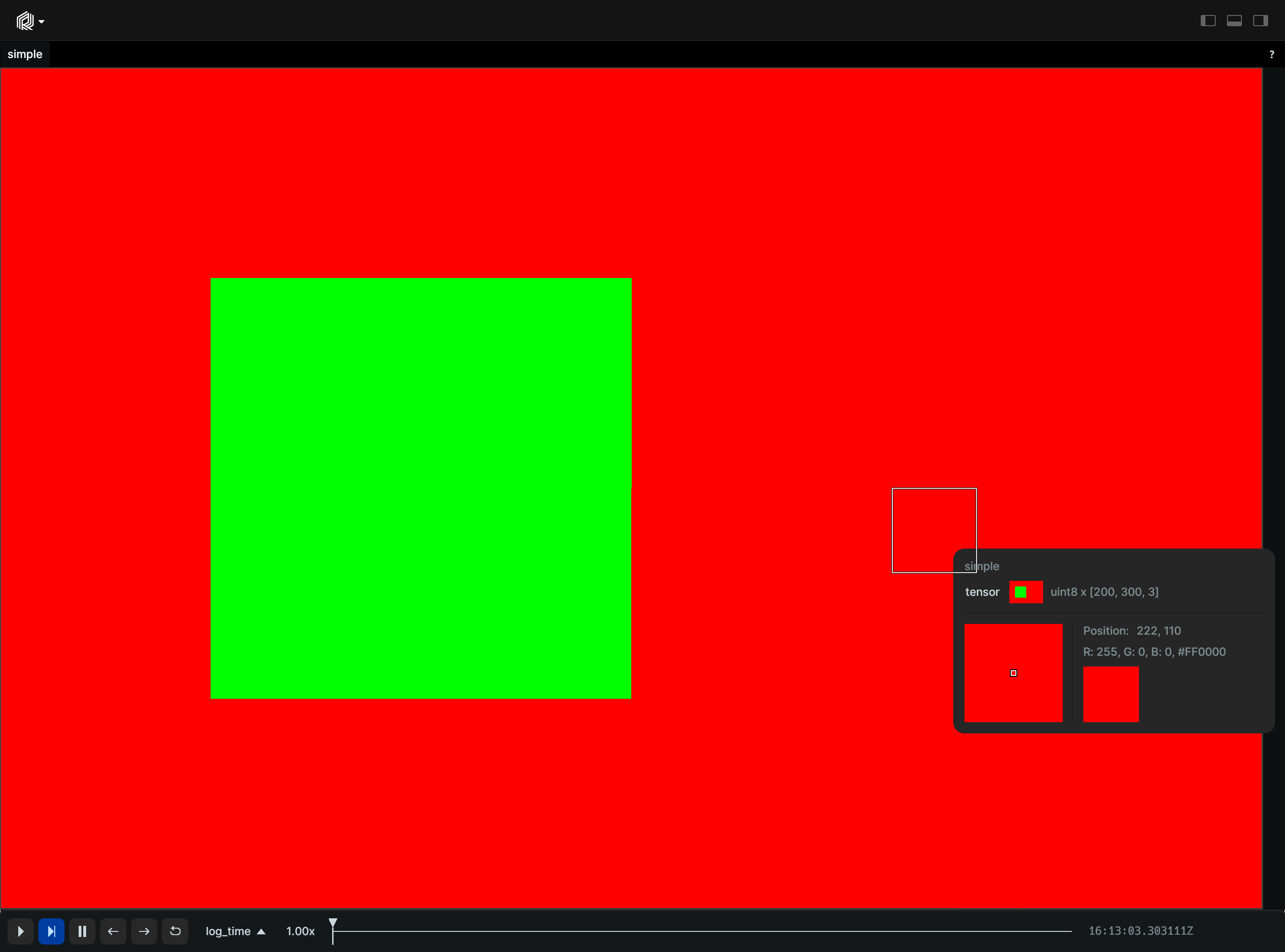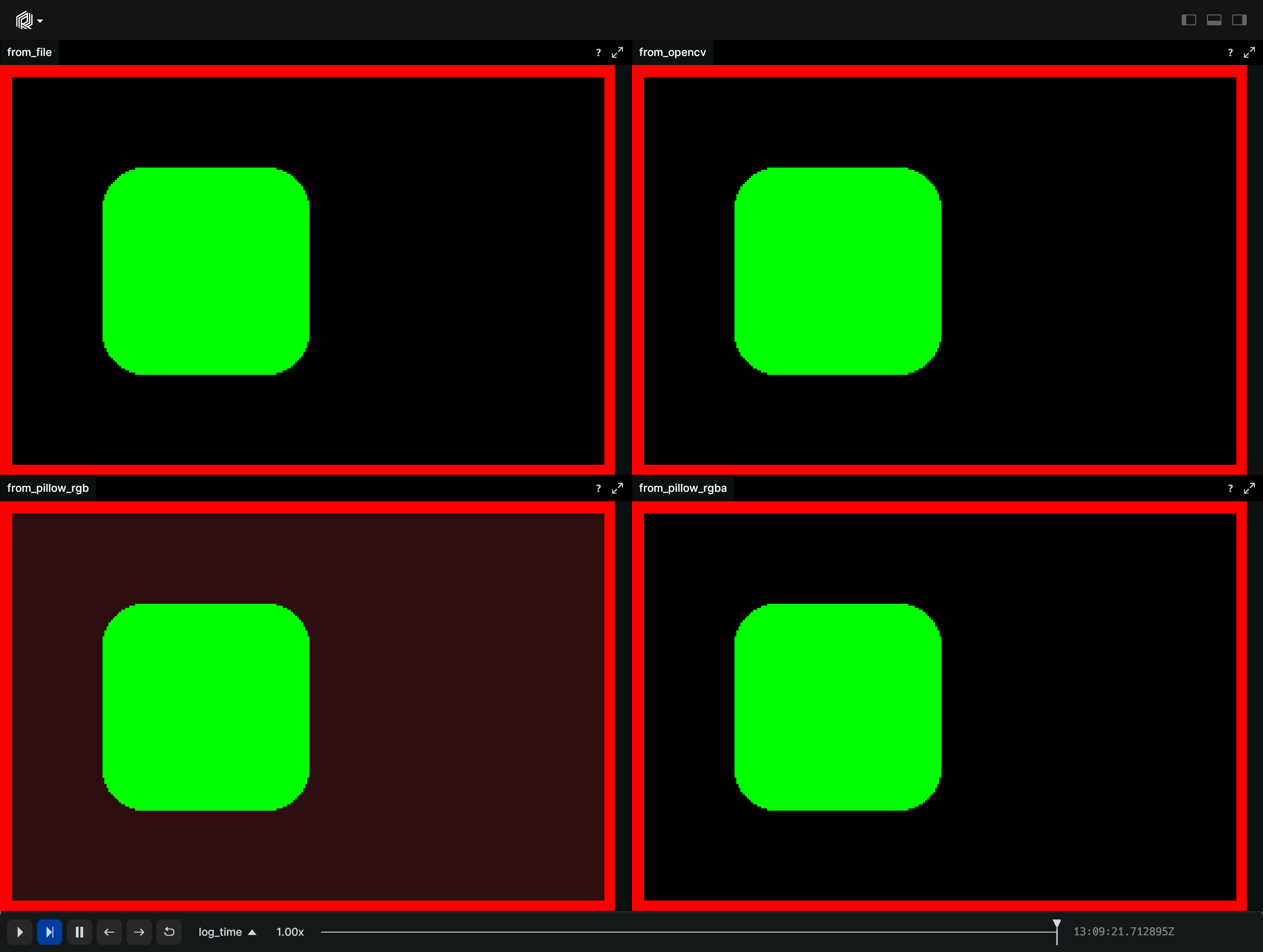# Image

`Image` represents a 2D raster image with various pixel format. They are a special case of 2D Tensor with an optional 3rd dimension when multiple color channels are used. Image with 1 (grayscale), 3 (RGB), or 4 (RGBA) channels are supported. Color channel maybe represented by any of the common scalar datatypes:

• `uint8`, `uint16`, `uint32`, `uint64`: Color channels in 0-`max_uint` sRGB gamma space, alpha in 0-`max_int` linear space.
• `float16`, `float32`, `float64`: Color channels in the 0.0-1.0 sRGB gamma space, alpha in 0.0-1.0 linear space.
• `int8`, `int16`, `int32`, `int64`: If all pixels are positive, they are interpreted as their unsigned counterparts. Otherwise, the image is normalized before display (the pixel with the lowest value is black and the pixel with the highest value is white).

The `colorrgba` component for the image applies a multiplicative tint.

## Components and APIs

Primary component: `tensor`

Secondary components: `colorrgba`, `draw_order`

Python APIs: log_image, log_image_file,

Rust API: Tensor

## Simple Example

``````"""Create and log an image."""

import numpy as np
import rerun as rr

# Create an image with Pillow
image = np.zeros((200, 300, 3), dtype=np.uint8)
image[:, :, 0] = 255
image[50:150, 50:150] = (0, 255, 0)

rr.init("rerun_example_images", spawn=True)

rr.log_image("simple", np.array(image))
````````````"""Log an image."""

import tempfile
from pathlib import Path

import cv2
import numpy as np
import rerun as rr
from PIL import Image, ImageDraw

# Save a transparent PNG to a temporary file.
_, file_path = tempfile.mkstemp(suffix=".png")
image = Image.new("RGBA", (300, 200), color=(0, 0, 0, 0))
draw = ImageDraw.Draw(image)
draw.rectangle((0, 0, 300, 200), outline=(255, 0, 0), width=6)
draw.rounded_rectangle((50, 50, 150, 150), fill=(0, 255, 0), radius=20)
image.save(file_path)

# Log the image from the file.
rr.log_image_file("from_file", img_path=Path(file_path))

# Read with Pillow and NumPy, and log the image.
image = np.array(Image.open(file_path))
rr.log_image("from_pillow_rgba", image)

# Convert to RGB, fill transparent pixels with a color, and log the image.
image_rgb = image[..., :3]
image_rgb[image[:, :, 3] == 0] = (45, 15, 15)
rr.log_image("from_pillow_rgb", image_rgb)